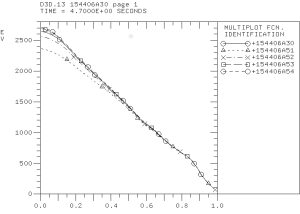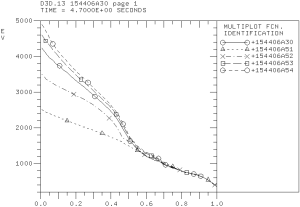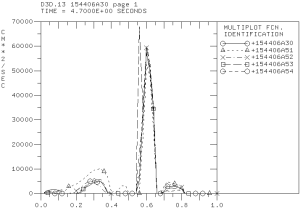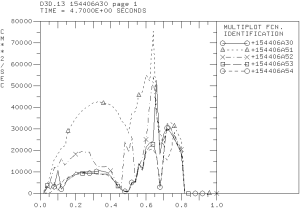## Shafranov shift scaling study for the DIII-D discharge 154406

The Shafranov shift effect is included through a geometric factor modifying the ion magnetic drift frequency, which varies strongly around each magnetic surface:$\omega_{Dj}=\displaystyle{\frac{-2k_{\theta}T_j}{Z_j e B R}} \left(\cos\theta+s\theta\sin\theta+\alpha_m\sin^2\theta\right)$

j is the ion specie index, Z is the ion charge,$k_{\theta}$ is the wave vector in the poloidal direction, s is the magnetic shear, and$\alpha_m$ is the alpha MHD which is defined as$\alpha_m=\displaystyle{\frac{2\mu_0 R q^2}{B^2}} \frac{dp}{dr}$.

The alpha MHD is proportional to the Shafranov shift and the effect of the Shafranov shift can be investigated by introducing a scaling factor for the last term in the first equation above:$\omega_{Dj}=\displaystyle{\frac{-2k_{\theta}T_j}{Z_j e B R}} \left(\cos\theta+s\theta\sin\theta+C_{\rm MMM}^{(4)}\alpha_m\sin^2\theta\right)$.

The coefficient$C_{\rm MMM}^{(4)}$ is added to the PTRANSP namelist. Five values of$C_{\rm MMM}^{(4)}$ are tested:$C_{\rm MMM}^{(4)} = 0.0$ – simulation 154406A51 (dashed curve with triangles)$C_{\rm MMM}^{(4)} = 0.5$ – simulation 154406A52 (dash-dotted curve with crosses)$C_{\rm MMM}^{(4)} = 1.0$ – simulation 154406A30 (solid curve with circles)$C_{\rm MMM}^{(4)} = 2.0$ – simulation 154406A53 (dashed curve with squares)$C_{\rm MMM}^{(4)} = 4.0$ – simulation 154406A54 (dashed curve with circles)The predicted electron (left) and ion (right) temperature profiles.Electron (left) and ion (right) thermal diffusivity profiles computed using the Weiland component of MMM7.1.

The Shafranov shift has stabilizing effect on electron and ion channels of transport. However, the effect on the electron channel is somewhat weaker. Also, the large spike in transport in the ITB region in the electron thermal diffusivities is found weakly affected by the Shafranov shift.